# pygimli.viewer.mpl¶

Matplotlib drawing functions used by pygimli.viewer.

## Overview¶

Functions

 addCoverageAlpha(patches, coverage[, …]) Add alpha values to the colors of a polygon collection. Set some common default properties for an axe. autolevel(z, nLevs[, logScale, zMin, zMax]) Create nLevs bins for the data array z based on matplotlib ticker. cacheFileName(fullname, vendor) Createfilename and path to cache download data. cmapFromName([cmapname, ncols, bad]) Get a colormap either from name or from keyworld list. createAnimation(fig, animate, nFrames, dpi, out) Create animation for the content of a given matplotlib figure. createColorBar(gci[, orientation, size, pad]) Create a Colorbar. createColorBarOnly([cMin, cMax, logScale, …]) Create figure with a colorbar. createMeshPatches(ax, mesh[, rasterized, …]) Utility function to create 2d mesh patches within a given ax. Generate triangle objects for later drawing. Utility function to create (or return existing) twin x axes for ax. Utility function to create (or return existing) twin x axes for ax. create_legend(ax, cmap, ids, classes) Create a list of patch objects that can be used for borehole legends. deg2MapTile(lon_deg, lat_deg, zoom) TODO Documentme. draw1DColumn(ax, x, val, thk[, width, …]) Draw a 1D column (e.g., from a 1D inversion) on a given ax. draw1dmodel(x[, thk, xlab, zlab, islog, z0]) DEPRECATED. draw1dmodelErr(x, xL[, xU, thk, xcol, ycol]) TODO. draw1dmodelLU(x, xL, xU[, thk]) Draw 1d model with lower and upper bounds. drawBlockMatrix(ax, mat, **kwargs) Draw a view of a matrix into the axes. drawBoundaryMarkers(ax, mesh[, …]) Draw boundary markers for mesh.boundaries with marker != 0 drawDataMatrix(ax, mat[, xmap, ymap, cMin, …]) Draw previously generated (generateVecMatrix) matrix. drawField(ax, mesh[, data, levels, nLevs, …]) Draw mesh with scalar field data. drawMesh(ax, mesh[, fitView]) Draw a 2d mesh into a given ax. drawMeshBoundaries(ax, mesh[, hideMesh, …]) Draw mesh on ax with boundary conditions colorized. drawModel(ax, mesh[, data, tri, rasterized, …]) Draw a 2d mesh and color the cell by the data. drawModel1D(ax[, thickness, values, model, …]) Draw 1d block model into axis ax. drawPLC(ax, mesh[, fillRegion, …]) Draw 2D PLC into given axes. drawParameterConstraints(ax, mesh, cMat[, …]) Draw inter parameter constraints between cells. drawSelectedMeshBoundaries(ax, boundaries[, …]) Draw mesh boundaries into a given axes. drawSelectedMeshBoundariesShadow(ax, boundaries) Draw mesh boundaries as shadows into a given axes. drawSensorAsMarker(ax, data) Draw Sensor marker, these marker are pickable. drawSensors(ax, sensors[, diam, coords]) Draw sensor positions as black dots with a given diameter. drawSparseMatrix(ax, mat, **kwargs) Draw a view of a matrix into the axes. drawStreamLines(ax, mesh, u[, nx, ny]) Draw streamlines for the gradients of field values u on a mesh. drawStreams(ax, mesh, data[, startStream, …]) Draw streamlines based on an unstructured mesh. drawValMapPatches(ax, vals[, xVec, yVec, dx, dy]) drawVecMatrix(ax, xvec, yvec, vals[, full]) findAndMaskBestClim(dataIn[, cMin, cMax, …]) TODO Documentme. generateMatrix(xvec, yvec, vals, **kwargs) Generate a data matrix from x/y and value vectors. getMapTile(xtile, ytile, zoom[, vendor, verbose]) Get a map tile from public mapping server. hold([val]) TODO WRITEME. insertUnitAtNextLastTick(ax, unit[, xlabel, …]) Replace the last-but-one tick label by unit symbol. mapTile2deg(xtile, ytile, zoom) Calculate the NW-corner of the square. patchMatrix(mat[, xmap, ymap, ax, cMin, …]) Plot previously generated (generateVecMatrix) matrix. patchValMap(vals[, xvec, yvec, ax, cMin, …]) Plot previously generated (generateVecMatrix) y map (category). plotDataContainerAsMatrix(*args, **kwargs) DEPRECATED naming scheme plotLines(ax, line_filename[, linewidth, step]) Read lines from file and plot over model. plotMatrix(mat, *args, **kwargs) Naming conventions. plotVecMatrix(xvec, yvec, vals[, full]) DEPRECATED for nameing Switch signs of depth ticks to be positive saveAnimation(mesh, data, out[, vData, plc, …]) Create and save an animation for a given mesh with a set of field data. saveAxes(ax, filename[, adjust]) Save axes as pdf. saveFigure(fig, filename[, pdfTrim]) Save figure as pdf. setCbarLevels(cbar[, cMin, cMax, nLevs, levels]) Set colorbar levels given a number of levels and min/max values. setMappableData(mappable, dataIn[, cMin, …]) Change the data values for a given mappable. setOutputStyle([dim, paperMargin, xScale, …]) Set preferred output style. setPlotStuff([fontsize, dpi]) TODO merge with setOutputStyle. show1dmodel(x[, thk, xlab, zlab, islog, z0]) Show 1d block model defined by value and thickness vectors. showDataContainerAsMatrix(data[, x, y, v]) Plot data container as matrix (cross-plot). showDataMatrix(mat[, xmap, ymap]) Show value map as matrix. showStitchedModels(models[, ax, x, cMin, …]) Show several 1d block models as (stitched) section. showValMapPatches(vals[, xVec, yVec, dx, dy]) showVecMatrix(xvec, yvec, vals[, full]) Plot three vectors as matrix. showfdemsounding(freq, inphase, quadrat[, …]) Show FDEM sounding as real(inphase) and imaginary (quadrature) fields. showmymatrix(mat, x, y[, dx, dy, xlab, …]) What is this good for?. twin(ax) Return the twin of ax if exist. underlayBKGMap(ax[, mode, utmzone, epsg, …]) Underlay digital orthophoto or topographic (mode=’DTK’) map under axes. underlayMap(ax, proj[, vendor, zoom, …]) Get a map from public mapping server and underlay it on the given ax. updateAxes(ax[, force]) For internal use. updateColorBar(cbar[, gci, cMin, cMax, …]) Update colorbar values. updateFig(fig[, force, sleep]) For internal use. wait(**kwargs) TODO WRITEME.

Classes

 BoreHole(fname) Class for handling (structural) borehole data for inclusion in plots. BoreHoles(fnames) Class to load and handle several boreholes belonging to one profile. CellBrowser(mesh[, data, ax]) Interactive cell browser on current or specified ax for a given mesh.

## Functions¶

### addCoverageAlpha¶

pygimli.viewer.mpl.addCoverageAlpha(patches, coverage, dropThreshold=0.4)[source]

Add alpha values to the colors of a polygon collection.

Parameters
• patches (2D mpl mappable) –

• coverage (array) – coverage values. Maximum coverage mean no opaqueness.

• dropThreshold (float) – relative minimum coverage

### adjustWorldAxes¶

pygimli.viewer.mpl.adjustWorldAxes(ax)[source]

Set some common default properties for an axe.

### autolevel¶

pygimli.viewer.mpl.autolevel(z, nLevs, logScale=None, zMin=None, zMax=None)[source]

Create nLevs bins for the data array z based on matplotlib ticker.

Examples

>>> import numpy as np
>>> from pygimli.viewer.mpl import autolevel
>>> x = np.linspace(1, 10, 100)
>>> autolevel(x, 3)
array([ 1. ,  5.5, 10. ])
>>> x = np.linspace(1, 1000, 100)
>>> autolevel(x, 4, logScale=True)
array([   1.,   10.,  100., 1000.])


### cacheFileName¶

pygimli.viewer.mpl.cacheFileName(fullname, vendor)[source]

Createfilename and path to cache download data.

### cmapFromName¶

pygimli.viewer.mpl.cmapFromName(cmapname='jet', ncols=256, bad=None, **kwargs)[source]

Get a colormap either from name or from keyworld list.

Parameters
• cmapname (str) – Name for the colormap.

• ncols (int) – Amount of colors.

• bad ([r,g,b,a]) – Default color for bad values [nan, inf] [white]

• kwargs (**) –

cMapstr

Name for the colormap

cmapstr

colormap name (old)

Returns

matplotlib Colormap

Return type

cMap

### createAnimation¶

pygimli.viewer.mpl.createAnimation(fig, animate, nFrames, dpi, out)[source]

Create animation for the content of a given matplotlib figure.

Until I know a better place.

### createColorBar¶

pygimli.viewer.mpl.createColorBar(gci, orientation='horizontal', size=0.2, pad=None, **kwargs)[source]

Create a Colorbar.

Shortcut to create a matplotlib colorbar within the ax for a given patchset. The colorbar is stored in the axes object as __cBar__ to avoid duplicates.

Parameters
• gci (matplotlib graphical instance) –

• orientation (string) –

• size (float) –

• pad (float) –

• **kwargs – Forwarded to updateColorBar

### createColorBarOnly¶

pygimli.viewer.mpl.createColorBarOnly(cMin=1, cMax=100, logScale=False, cMap=None, nLevs=5, label=None, orientation='horizontal', savefig=None, ax=None, **kwargs)[source]

Create figure with a colorbar.

Create figure with a colorbar.

Parameters

**kwargs – Forwarded to mpl.colorbar.ColorbarBase.

Returns

The created figure.

Return type

fig

Examples

>>> # import pygimli as pg
>>> # from pygimli.viewer.mpl import createColorBarOnly
>>> # createColorBarOnly(cMin=0.2, cMax=5, logScale=False,
>>> #                   cMap='b2r',
>>> #                   nLevs=7,
>>> #                   label=r'Ratio',
>>> #                   orientation='horizontal')
>>> # pg.wait()


### createMeshPatches¶

pygimli.viewer.mpl.createMeshPatches(ax, mesh, rasterized=False, verbose=True)[source]

Utility function to create 2d mesh patches within a given ax.

### createTriangles¶

pygimli.viewer.mpl.createTriangles(mesh)[source]

Generate triangle objects for later drawing.

Creates triangle for each 2D triangle cell or 3D boundary. Quads will be split into two triangles. Result will be cached into mesh._triData.

Parameters

mesh (GIMLI::Mesh) – 2D mesh or 3D mesh

Returns

• x (numpy array) – x position of nodes

• y (numpy array) – x position of nodes

• triangles (numpy array Cx3) – cell indices for each triangle, quad or boundary face

• z (numpy array) – z position for given indices

• dataIdx (list of int) – List of indices for a data array

### createTwinX¶

pygimli.viewer.mpl.createTwinX(ax)[source]

Utility function to create (or return existing) twin x axes for ax.

### createTwinY¶

pygimli.viewer.mpl.createTwinY(ax)[source]

Utility function to create (or return existing) twin x axes for ax.

### create_legend¶

pygimli.viewer.mpl.create_legend(ax, cmap, ids, classes)[source]

Create a list of patch objects that can be used for borehole legends.

### deg2MapTile¶

pygimli.viewer.mpl.deg2MapTile(lon_deg, lat_deg, zoom)[source]

TODO Documentme.

### draw1DColumn¶

pygimli.viewer.mpl.draw1DColumn(ax, x, val, thk, width=30, ztopo=0, cmin=1, cmax=1000, cmap=None, name=None, textoffset=0.0)[source]

Draw a 1D column (e.g., from a 1D inversion) on a given ax.

>>> import numpy as np
>>> import matplotlib.pyplot as plt
>>> from pygimli.viewer.mpl import draw1DColumn
>>> thk = [1, 2, 3, 4]
>>> val = thk
>>> fig, ax = plt.subplots()
>>> draw1DColumn(ax, 0.5, val, thk, width=0.1, cmin=1, cmax=4, name="VES")
<matplotlib.collections.PatchCollection object at ...>
>>> _ = ax.set_ylim(-np.sum(thk), 0)


(png, pdf)### draw1dmodel¶

pygimli.viewer.mpl.draw1dmodel(x, thk=None, xlab=None, zlab='z in m', islog=True, z0=0)[source]

DEPRECATED.

### draw1dmodelErr¶

pygimli.viewer.mpl.draw1dmodelErr(x, xL, xU=None, thk=None, xcol='g', ycol='r', **kwargs)[source]

TODO.

### draw1dmodelLU¶

pygimli.viewer.mpl.draw1dmodelLU(x, xL, xU, thk=None, **kwargs)[source]

Draw 1d model with lower and upper bounds.

### drawBlockMatrix¶

pygimli.viewer.mpl.drawBlockMatrix(ax, mat, **kwargs)[source]

Draw a view of a matrix into the axes.

Parameters
• ax (mpl axis instance, optional) – Axis instance where the matrix will be plotted.

• mat (pg.Matrix.BlockMatrix) –

Keyword Arguments

spy (bool [False]) – Draw all matrix entries instead of colored blocks

Returns

Return type

ax

>>> import numpy as np
>>> import pygimli as pg
>>> I = pg.matrix.IdentityMatrix(10)
>>> SM = pg.matrix.SparseMapMatrix()
>>> for i in range(10):
...     SM.setVal(i, 10 - i, 5.0)
...     SM.setVal(i,  i, 5.0)
>>> B = pg.matrix.BlockMatrix()
>>> B.add(I, 0, 0)
0
>>> B.add(SM, 10, 10)
1
>>> print(B)
pg.matrix.BlockMatrix of size 20 x 21 consisting of 2 submatrices.
>>> fig, (ax1, ax2) = pg.plt.subplots(1, 2, sharey=True)
>>> _ = pg.show(B, ax=ax1)
>>> _ = pg.show(B, spy=True, ax=ax2)


(png, pdf)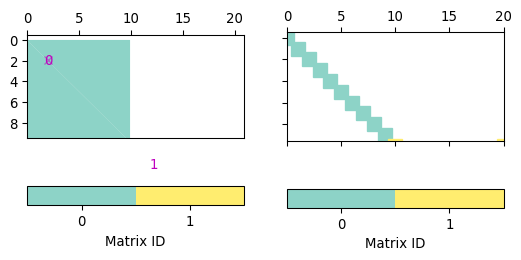### drawBoundaryMarkers¶

pygimli.viewer.mpl.drawBoundaryMarkers(ax, mesh, clipBoundaryMarkers=False, **kwargs)[source]

Draw boundary markers for mesh.boundaries with marker != 0

Parameters
• mesh (GIMLI::Mesh) – Mesh that have the boundary markers.

• clipBoundaryMarkers (bool [False]) – Clip boundary marker to the axes limits if needed.

Keyword Arguments

**kwargs – Forwarded to plot

>>> import pygimli as pg
>>> import pygimli.meshtools as mt
>>> c0 = mt.createCircle(pos=(0.0, 0.0), radius=1, segments=4)
>>> l0 = mt.createPolygon([[-0.5, 0.0], [.5, 0.0]], boundaryMarker=2)
>>> l1 = mt.createPolygon([[-0.25, -0.25], [0.0, -0.5], [0.25, -0.25]],
...                       interpolate='spline', addNodes=4,
...                       boundaryMarker=3)
>>> l2 = mt.createPolygon([[-0.25, 0.25], [0.0, 0.5], [0.25, 0.25]],
...                       interpolate='spline', addNodes=4,
...                       isClosed=True, boundaryMarker=3)
>>> mesh = mt.createMesh([c0, l0, l1, l2], area=0.01)
>>> ax, _ = pg.show(mesh)
>>> pg.viewer.mpl.drawBoundaryMarkers(ax, mesh)


(png, pdf)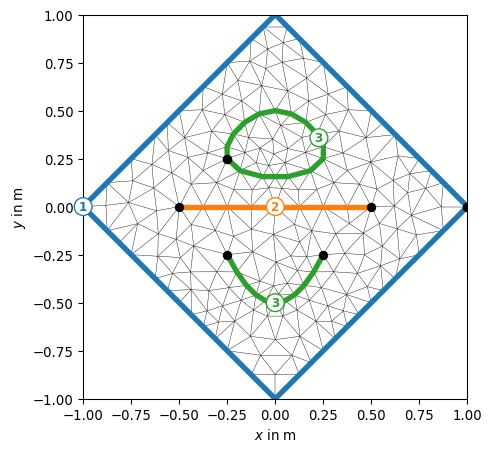### drawDataMatrix¶

pygimli.viewer.mpl.drawDataMatrix(ax, mat, xmap=None, ymap=None, cMin=None, cMax=None, logScale=None, label=None, **kwargs)[source]

Draw previously generated (generateVecMatrix) matrix.

Parameters
• ax (mpl.axis) – axis to plot, if not given a new figure is created

• mat (numpy.array2d) – matrix to show

• xmap (dict {i:num}) – dict (must match A.shape)

• ymap (iterable) – vector for x axis (must match A.shape)

• cMin/cMax (float) – minimum/maximum color values

• logScale (bool) – logarithmic colour scale [min(A)>0]

• label (string) – colorbar label

### drawField¶

pygimli.viewer.mpl.drawField(ax, mesh, data=None, levels=None, nLevs=5, cMin=None, cMax=None, nCols=None, logScale=False, fitView=True, **kwargs)[source]

Draw mesh with scalar field data.

Draw scalar field into MPL axes using matplotlib triplot. Only for triangle/quadrangle meshes currently

Parameters
• ax (mpl axe) –

• mesh (GIMLI::Mesh) – 2D mesh

• data (iterable) – Scalar field values. Can be of length mesh.cellCount() or mesh.nodeCount().

• levels (iterable of type float) – Values for contour lines. If empty auto generated from nLevs.

• nLevs (int) – Number of contour levels based on cMin, cMax and logScale.

• cMin (float [None]) – Minimal contour value. If None min(data).

• cMax (float [None]) – Maximal contour value. If None max(data).

• logScale (bool [False]) – Levels and colors distributes with logarithmic scale.

• fitView (bool [True]) – Adjust ax limits to mesh bounding box.

Keyword Arguments
• shading ('flat' | 'gouraud') –

• fillContour ([True]) –

• contourLines ([True]) –

• **kwargs – Additional kwargs forwarded to ax.tripcolor, ax.tricontour, ax.tricontourf

Returns

gci – The current image object useful for post color scaling

Return type

image object

>>> import numpy as np
>>> import matplotlib.pyplot as plt
>>> import pygimli as pg
>>> from pygimli.viewer.mpl import drawField
>>> n = np.linspace(0, -2, 11)
>>> mesh = pg.createGrid(x=n, y=n)
>>> nx = pg.x(mesh.positions())
>>> ny = pg.y(mesh.positions())
>>> data = np.cos(1.5 * nx) * np.sin(1.5 * ny)
>>> fig, ax = plt.subplots()
>>> drawField(ax, mesh, data)
<matplotlib.tri.tricontour.TriContourSet ...>


(png, pdf)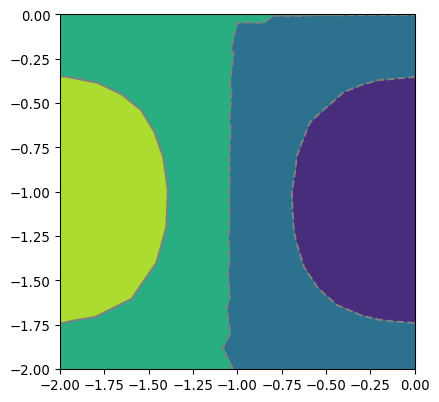### drawMesh¶

pygimli.viewer.mpl.drawMesh(ax, mesh, fitView=True, **kwargs)[source]

Draw a 2d mesh into a given ax.

Set the limits of the ax tor the mesh extent.

Parameters
• ax (mpl axe instance) – Axis instance where the mesh is plotted.

• mesh (GIMLI::Mesh) – The 2D mesh which will be drawn.

• fitView (bool [True]) – Adjust ax limits to mesh bounding box.

Keyword Arguments
• **kwargs – Additional kwargs forward to drawPLC or drawMeshBoundaries.

• %(drawMeshBoundaries)

• %(drawPLC)

>>> import numpy as np
>>> import matplotlib.pyplot as plt
>>> import pygimli as pg
>>> from pygimli.viewer.mpl import drawMesh
>>> n = np.linspace(1, 2, 10)
>>> mesh = pg.createGrid(x=n, y=n)
>>> fig, ax = plt.subplots()
>>> drawMesh(ax, mesh)
>>> plt.show()


(png, pdf)Examples using pygimli.viewer.mpl.drawMesh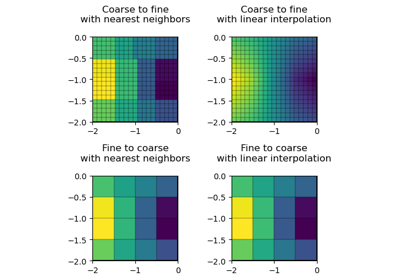### drawMeshBoundaries¶

pygimli.viewer.mpl.drawMeshBoundaries(ax, mesh, hideMesh=False, useColorMap=False, fitView=True, lw=None, color=None, **kwargs)[source]

Draw mesh on ax with boundary conditions colorized.

Parameters
• mesh (GIMLI::Mesh) –

• hideMesh (bool [False]) – Show only the boundary of the mesh and omit inner edges that separate the cells.

• useColorMap (bool[False]) – Apply the default colormap to boundaries with marker values > 0

• fitView (bool [True]) – Adjust ax limits to mesh bounding box.

• lw (float [None]) – Linewidth. When set to None then lw depends on boundary marker. Linewidth [0.3] for edges with marker == 0 if hideMesh is False.

• color (None) – Color for special lines. If set to None automatic “black”.

>>> import numpy as np
>>> import matplotlib.pyplot as plt
>>> import pygimli as pg
>>> from pygimli.viewer.mpl import drawMeshBoundaries
>>> n = np.linspace(0, -2, 11)
>>> mesh = pg.createGrid(x=n, y=n)
>>> for bound in mesh.boundaries():
...     if not bound.rightCell():
...         bound.setMarker(pg.core.MARKER_BOUND_MIXED)
...     if bound.center().y() == 0:
...         bound.setMarker(pg.core.MARKER_BOUND_HOMOGEN_NEUMANN)
>>> fig, ax = plt.subplots()
>>> drawMeshBoundaries(ax, mesh)


(png, pdf)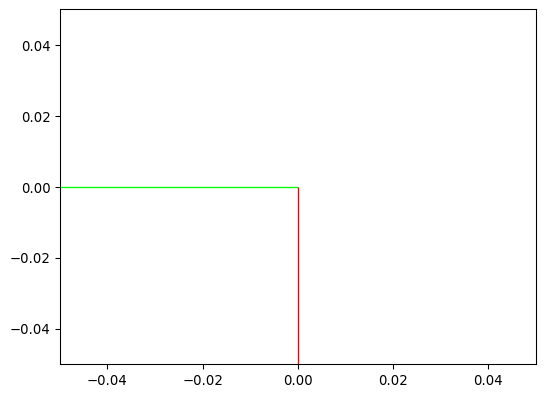### drawModel¶

pygimli.viewer.mpl.drawModel(ax, mesh, data=None, tri=False, rasterized=False, cMin=None, cMax=None, logScale=False, xlabel=None, ylabel=None, fitView=True, verbose=False, **kwargs)[source]

Draw a 2d mesh and color the cell by the data.

Parameters
• ax (mpl axis instance, optional) – Axis instance where the mesh is plotted (default is current axis).

• mesh (GIMLI::Mesh) – The plotted mesh to browse through.

• data (array, optional) – Data to draw. Should either equal numbers of cells or nodes of the corresponding mesh.

• tri (boolean, optional) – use MPL tripcolor (experimental)

• rasterized (boolean, optional) – Rasterize mesh patches to reduce file size and avoid zooming artifacts in some PDF viewers.

• fitView (bool [True]) – Adjust ax limits to mesh bounding box.

Keyword Arguments

**kwargs – Additional kwargs forwarded to the draw functions and mpl methods, respectively.

Returns

gci

Return type

matplotlib graphics object

>>> import numpy as np
>>> import matplotlib.pyplot as plt
>>> import pygimli as pg
>>> from pygimli.viewer.mpl import drawModel
>>> n = np.linspace(0, -2, 11)
>>> mesh = pg.createGrid(x=n, y=n)
>>> mx = pg.x(mesh.cellCenter())
>>> my = pg.y(mesh.cellCenter())
>>> data = np.cos(1.5 * mx) * np.sin(1.5 * my)
>>> fig, ax = plt.subplots()
>>> drawModel(ax, mesh, data)
<matplotlib.collections.PolyCollection object at ...>


(png, pdf)Examples using pygimli.viewer.mpl.drawModel### drawModel1D¶

pygimli.viewer.mpl.drawModel1D(ax, thickness=None, values=None, model=None, depths=None, plot='plot', xlabel='Resistivity $(\\Omega$m$)$', zlabel='Depth (m)', z0=0, **kwargs)[source]

Draw 1d block model into axis ax.

Draw 1d block model into axis ax defined by values and thickness vectors using plot function. For log y cases, z0 should be set > 0 so that the default becomes 1.

Parameters
• ax (mpl axes) – Matplotlib Axes object to plot into.

• values (iterable [float]) – [N] Values for each layer plus lower background.

• thickness (iterable [float]) – [N-1] thickness for each layer. Either thickness or depths must be set.

• depths (iterable [float]) – [N-1] Values for layer depths (positive z-coordinates). Either thickness or depths must be set.

• model (iterable [float]) – Shortcut to use default model definition. thks = model[0:nLay] values = model[nLay:]

• plot (string) – Matplotlib plotting function. ‘plot’, ‘semilogx’, ‘semilogy’, ‘loglog’

• xlabel (str) – Label for x axis.

• ylabel (str) – Label for y axis.

• z0 (float) – Starting depth in m

• **kwargs (dict()) – Forwarded to the plot routine

>>> import matplotlib.pyplot as plt
>>> import numpy as np
>>> import pygimli as pg
>>> # plt.style.use('ggplot')
>>> thk = [1, 4, 4]
>>> res = np.array([10., 5, 15, 50])
>>> fig, ax = plt.subplots()
>>> pg.viewer.mpl.drawModel1D(ax, values=res*5, depths=np.cumsum(thk),
...                          plot='semilogx', color='blue')
>>> pg.viewer.mpl.drawModel1D(ax, values=res, thickness=thk, z0=1,
...                          plot='semilogx', color='red')
>>> pg.wait()


(png, pdf)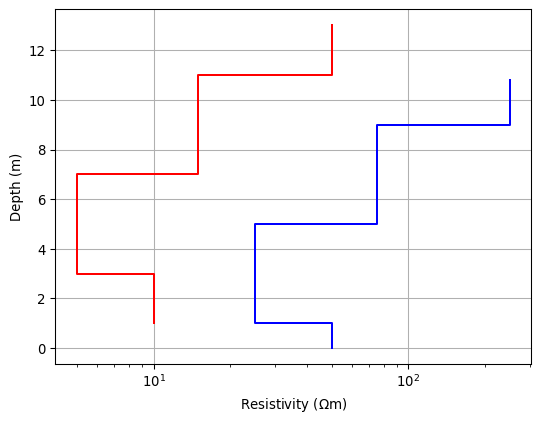Examples using pygimli.viewer.mpl.drawModel1D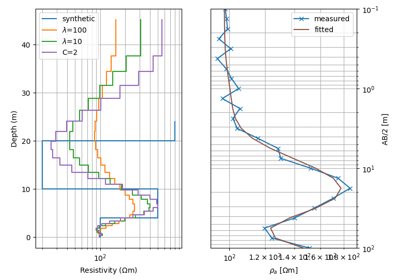### drawPLC¶

pygimli.viewer.mpl.drawPLC(ax, mesh, fillRegion=True, regionMarker=True, boundaryMarkers=False, showNodes=False, fitView=True, **kwargs)[source]

Draw 2D PLC into given axes.

Parameters
• ax (mpl axe) –

• mesh (GIMLI::Mesh) –

• fillRegion (bool [True]) – Fill the regions with default colormap.

• regionMarker (bool [True]) – Show region marker.

• boundaryMarkers (bool [False]) – Show boundary marker.

• showNodes (bool [False]) – Draw all nodes as little dots.

• fitView (bool [True]) – Adjust ax limits to mesh bounding box.

Keyword Arguments

**kwargs – Additional kwargs forwarded to the draw functions and mpl methods, respectively.

>>> import matplotlib.pyplot as plt
>>> import pygimli as pg
>>> import pygimli.meshtools as mt
>>> # Create geometry definition for the modelling domain
>>> world = mt.createWorld(start=[-20, 0], end=[20, -16],
...                        layers=[-2, -8], worldMarker=False)
>>> # Create a heterogeneous block
>>> block = mt.createRectangle(start=[-6, -3.5], end=[6, -6.0],
...                            marker=10,  boundaryMarker=10, area=0.1)
>>> fig, ax = plt.subplots()
>>> geom = world + block
>>> _ = pg.viewer.mpl.drawPLC(ax, geom)


(png, pdf)### drawParameterConstraints¶

pygimli.viewer.mpl.drawParameterConstraints(ax, mesh, cMat, cWeights=None)[source]

Draw inter parameter constraints between cells.

Parameters
• ax (MPL axes) –

• mesh (GIMLI::Mesh) – 2d mesh

• cMat (GIMLI::SparseMapMatrix) – ConstraintsMatrix

• cWeights (iterable float) – Weights for all constraints. Need to have a lengths == cMat.rows()

### drawSelectedMeshBoundaries¶

pygimli.viewer.mpl.drawSelectedMeshBoundaries(ax, boundaries, color=None, linewidth=1.0, linestyles='-')[source]

Draw mesh boundaries into a given axes.

Parameters
• ax (matplotlib axes) – axes to plot into

• boundaries (GIMLI::Mesh boundary vector) – collection of boundaries to plot

• color (matplotlib color |str [None]) – matching color or string, else colors are according to markers

• linewidth (float [1.0]) – line width

• linestyles (linestyle for line collection, i.e. solid or dashed) –

Returns

lco

Return type

matplotlib line collection object

Examples using pygimli.viewer.mpl.drawSelectedMeshBoundaries### drawSelectedMeshBoundariesShadow¶

pygimli.viewer.mpl.drawSelectedMeshBoundariesShadow(ax, boundaries, first='x', second='y', color=0.3, 0.3, 0.3, 1.0)[source]

Draw mesh boundaries as shadows into a given axes.

Parameters
• ax (matplotlib axes) – axes to plot into

• boundaries (GIMLI::Mesh boundary vector) – collection of boundaries to plot

• / second (first) – attribute names to retrieve from nodes

• color (matplotlib color |str [None]) – matching color or string, else colors are according to markers

• linewidth (float [1.0]) – line width

Returns

lco

Return type

matplotlib line collection object

### drawSensorAsMarker¶

pygimli.viewer.mpl.drawSensorAsMarker(ax, data)[source]

Draw Sensor marker, these marker are pickable.

### drawSensors¶

pygimli.viewer.mpl.drawSensors(ax, sensors, diam=None, coords=None, **kwargs)[source]

Draw sensor positions as black dots with a given diameter.

Parameters
• ax (mpl axe instance) –

• sensors (vector or list of RVector3) – list of positions to plot

• diam (float [None]) – diameter of circles (None leads to point distance by 8)

• coords ((int, int) [0, 1]) – Coordinates to take (usually x and y)

Keyword Arguments

**kwargs – Additional kwargs forwarded to mpl.PatchCollection, mpl.Circle

>>> import numpy as np
>>> import matplotlib.pyplot as plt
>>> import pygimli as pg
>>> from pygimli.viewer.mpl import drawSensors
>>> sensors = np.random.rand(5, 2)
>>> fig, ax = pg.plt.subplots()
>>> drawSensors(ax, sensors, diam=0.02, coords=[0, 1])
>>> ax.set_aspect('equal')
>>> pg.wait()


(png, pdf)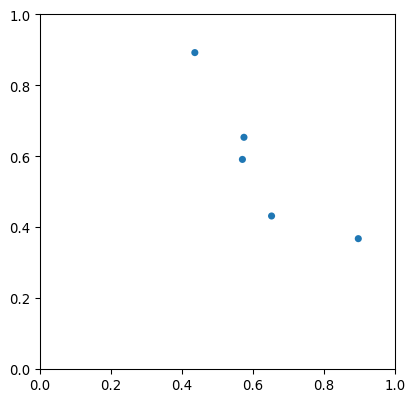Examples using pygimli.viewer.mpl.drawSensors### drawSparseMatrix¶

pygimli.viewer.mpl.drawSparseMatrix(ax, mat, **kwargs)[source]

Draw a view of a matrix into the axes.

Parameters
• ax (mpl axis instance, optional) – Axis instance where the matrix will be plotted.

• mat (pg.matrix.SparseMatrix or pg.matrix.SparseMapMatrix) –

Returns

Return type

mpl.lines.line2d

>>> import numpy as np
>>> import pygimli as pg
>>> from pygimli.viewer.mpl import drawSparseMatrix
>>> A = pg.randn((10,10), seed=0)
>>> SM = pg.core.SparseMapMatrix()
>>> for i in range(10):
...     SM.setVal(i, i, 5.0)
>>> fig, (ax1, ax2) = pg.plt.subplots(1, 2, sharey=True, sharex=True)
>>> _ = drawSparseMatrix(ax1, A, colOffset=5, rowOffset=5, color='blue')
>>> _ = drawSparseMatrix(ax2, SM, color='green')


(png, pdf)### drawStreamLines¶

pygimli.viewer.mpl.drawStreamLines(ax, mesh, u, nx=25, ny=25, **kwargs)[source]

Draw streamlines for the gradients of field values u on a mesh.

The matplotlib routine streamplot needs equidistant spacings so we interpolate first on a grid defined by nx and ny nodes. Additionally arguments are piped to streamplot.

This works only for rectangular regions. You should use pg.viewer.mpl.drawStreams, which is more comfortable and more flexible.

Parameters
• ax (mpl axe) –

• mesh (GIMLI::Mesh) – 2D mesh

• u (iterable float) – Scalar data field.

### drawStreams¶

pygimli.viewer.mpl.drawStreams(ax, mesh, data, startStream=3, coarseMesh=None, quiver=False, **kwargs)[source]

Draw streamlines based on an unstructured mesh.

Every cell contains only one streamline and every new stream line starts in the center of a cell. You can alternatively provide a second mesh with coarser mesh to draw streams for.

Parameters
• ax (matplotlib.ax) – ax to draw into

• mesh (GIMLI::Mesh) – 2d mesh

• data (iterable float | [float, float] | pg.core.R3Vector) – If data is an array (per cell or node) gradients are calculated otherwise the data will be interpreted as vector field per nodes or cell centers.

• startStream (int) – variate the start stream drawing, try values from 1 to 3 what every you like more.

• coarseMesh (GIMLI::Mesh) – Instead of draw a stream for every cell in mesh, draw a streamline segment for each cell in coarseMesh.

• quiver (bool [False]) – Draw arrows instead of streamlines.

Keyword Arguments

**kwargs – Additional kwargs forwarded to axe.quiver, drawStreamLine_

>>> import numpy as np
>>> import matplotlib.pyplot as plt
>>> import pygimli as pg
>>> from pygimli.viewer.mpl import drawStreams
>>> n = np.linspace(0, 1, 10)
>>> mesh = pg.createGrid(x=n, y=n)
>>> nx = pg.x(mesh.positions())
>>> ny = pg.y(mesh.positions())
>>> data = np.cos(1.5 * nx) * np.sin(1.5 * ny)
>>> fig, ax = plt.subplots()
>>> drawStreams(ax, mesh, data, color='red')
>>> drawStreams(ax, mesh, data, dropTol=0.9)
>>> drawStreams(ax, mesh, pg.solver.grad(mesh, data),
...             color='green', quiver=True)
>>> ax.set_aspect('equal')
>>> pg.wait()


(png, pdf)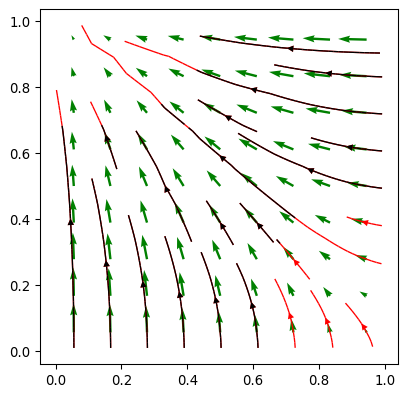Examples using pygimli.viewer.mpl.drawStreams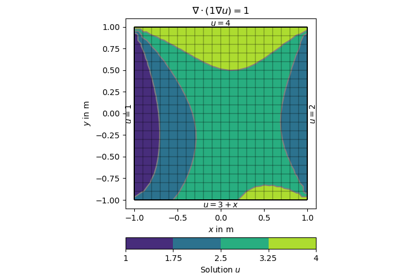### drawValMapPatches¶

pygimli.viewer.mpl.drawValMapPatches(ax, vals, xVec=None, yVec=None, dx=1, dy=None, **kwargs)[source]

### drawVecMatrix¶

pygimli.viewer.mpl.drawVecMatrix(ax, xvec, yvec, vals, full=False, **kwargs)[source]

### findAndMaskBestClim¶

pygimli.viewer.mpl.findAndMaskBestClim(dataIn, cMin=None, cMax=None, dropColLimitsPerc=5, logScale=False)[source]

TODO Documentme.

### generateMatrix¶

pygimli.viewer.mpl.generateMatrix(xvec, yvec, vals, **kwargs)[source]

Generate a data matrix from x/y and value vectors.

Parameters
• yvec, vals (xvec,) –

• full (bool [False]) – generate a fully symmetric matrix containing all unique xvec+yvec otherwise A is squeezed to the individual unique vectors

Returns

• A (np.ndarray(2d)) – matrix containing the values sorted according to unique xvec/yvec

• xmap/ymap (dict {key: num}) – dictionaries for accessing matrix position (row/col number from x/y[i])

### getMapTile¶

pygimli.viewer.mpl.getMapTile(xtile, ytile, zoom, vendor='OSM', verbose=False)[source]

Get a map tile from public mapping server.

Its not recommended to use the google maps tile server without the google maps api so if you use it to often your IP will be blacklisted

TODO: look here for more vendors https://github.com/Leaflet/Leaflet TODO: Try http://scitools.org.uk/cartopy/docs/v0.14/index.html TODO: Try https://github.com/jwass/mplleaflet

Parameters
• xtile (int) –

• ytile (int) –

• zoom (int) –

• vendor (str) – . ‘OSM’ or ‘Open Street Map’ (tile.openstreetmap.org) . ‘GM’ or ‘Google Maps’ (mt.google.com) (do not use it to much)

• verbose (bool [false]) – be verbose

### hold¶

pygimli.viewer.mpl.hold(val=1)[source]

TODO WRITEME.

### insertUnitAtNextLastTick¶

pygimli.viewer.mpl.insertUnitAtNextLastTick(ax, unit, xlabel=True, position=- 2)[source]

Replace the last-but-one tick label by unit symbol.

### mapTile2deg¶

pygimli.viewer.mpl.mapTile2deg(xtile, ytile, zoom)[source]

Calculate the NW-corner of the square.

Use the function with xtile+1 and/or ytile+1 to get the other corners. With xtile+0.5 ytile+0.5 it will return the center of the tile.

### patchMatrix¶

pygimli.viewer.mpl.patchMatrix(mat, xmap=None, ymap=None, ax=None, cMin=None, cMax=None, logScale=None, label=None, dx=1, **kwargs)[source]

Plot previously generated (generateVecMatrix) matrix.

Parameters
• mat (numpy.array2d) – matrix to show

• xmap (dict {i:num}) – dict (must match A.shape)

• ymap (iterable) – vector for x axis (must match A.shape)

• ax (mpl.axis) – axis to plot, if not given a new figure is created

• cMin/cMax (float) – minimum/maximum color values

• logScale (bool) – logarithmic colour scale [min(A)>0]

• label (string) – colorbar label

• dx (float) – width of the matrix elements (by default 1)

### patchValMap¶

pygimli.viewer.mpl.patchValMap(vals, xvec=None, yvec=None, ax=None, cMin=None, cMax=None, logScale=None, label=None, dx=1, dy=None, cTrim=0, **kwargs)[source]

Plot previously generated (generateVecMatrix) y map (category).

Parameters
• vals (iterable) – Data values to show.

• xvec (dict {i:num}) – dict (must match vals.shape)

• ymap (iterable) – vector for x axis (must match vals.shape)

• ax (mpl.axis) – axis to plot, if not given a new figure is created

• cMin/cMax (float) – minimum/maximum color values

• cTrim (float ) – use trim value to exclude outer cTrim percent of data from color scale

• logScale (bool) – logarithmic colour scale [min(vals)>0]

• label (string) – colorbar label

• kwargs (**) –

• circularbool

Plot in polar coordinates.

### plotDataContainerAsMatrix¶

pygimli.viewer.mpl.plotDataContainerAsMatrix(*args, **kwargs)[source]

DEPRECATED naming scheme

### plotLines¶

pygimli.viewer.mpl.plotLines(ax, line_filename, linewidth=1.0, step=1)[source]

Read lines from file and plot over model.

### plotMatrix¶

pygimli.viewer.mpl.plotMatrix(mat, *args, **kwargs)[source]

Naming conventions. Use drawDataMatrix or showDataMatrix

### plotVecMatrix¶

pygimli.viewer.mpl.plotVecMatrix(xvec, yvec, vals, full=False, **kwargs)[source]

DEPRECATED for nameing

### renameDepthTicks¶

pygimli.viewer.mpl.renameDepthTicks(ax)[source]

Switch signs of depth ticks to be positive

### saveAnimation¶

pygimli.viewer.mpl.saveAnimation(mesh, data, out, vData=None, plc=None, label='', cMin=None, cMax=None, logScale=False, cmap=None, **kwargs)[source]

Create and save an animation for a given mesh with a set of field data.

Until I know a better place.

### saveAxes¶

pygimli.viewer.mpl.saveAxes(ax, filename, adjust=False)[source]

Save axes as pdf.

### saveFigure¶

pygimli.viewer.mpl.saveFigure(fig, filename, pdfTrim=False)[source]

Save figure as pdf.

### setCbarLevels¶

pygimli.viewer.mpl.setCbarLevels(cbar, cMin=None, cMax=None, nLevs=5, levels=None)[source]

Set colorbar levels given a number of levels and min/max values.

### setMappableData¶

pygimli.viewer.mpl.setMappableData(mappable, dataIn, cMin=None, cMax=None, logScale=None, **kwargs)[source]

Change the data values for a given mappable.

### setOutputStyle¶

pygimli.viewer.mpl.setOutputStyle(dim='w', paperMargin=5, xScale=1.0, yScale=1.0, fontsize=9, scale=1, usetex=True)[source]

Set preferred output style.

### setPlotStuff¶

pygimli.viewer.mpl.setPlotStuff(fontsize=7, dpi=None)[source]

TODO merge with setOutputStyle.

Change ugly name.

### show1dmodel¶

pygimli.viewer.mpl.show1dmodel(x, thk=None, xlab=None, zlab='z in m', islog=True, z0=0, **kwargs)[source]

Show 1d block model defined by value and thickness vectors.

### showDataContainerAsMatrix¶

pygimli.viewer.mpl.showDataContainerAsMatrix(data, x=None, y=None, v=None, **kwargs)[source]

Plot data container as matrix (cross-plot).

for each x, y and v token strings or vectors should be given

### showDataMatrix¶

pygimli.viewer.mpl.showDataMatrix(mat, xmap=None, ymap=None, **kwargs)[source]

Show value map as matrix.

Returns

• ax (matplotlib axes object) – axes object

• cb (matplotlib colorbar) – colorbar object

### showStitchedModels¶

pygimli.viewer.mpl.showStitchedModels(models, ax=None, x=None, cMin=None, cMax=None, logScale=True, title=None, zMin=0, zMax=0, zLog=False, cmap='jet', **kwargs)[source]

Show several 1d block models as (stitched) section.

Parameters
• model (iterable of iterable (np.ndarray or list of np.array)) – 1D models (consisting of thicknesses and values) to plot

• ax (matplotlib axes [None - create new]) – axes object to plot in

• x (iterable) – positions of individual models

• cMin/cMax (float [None - autodetection from range]) – minimum and maximum colorscale range

• logScale (bool [True]) – use logarithmic color scaling

• zMin/zMax (float [0 - automatic]) – range for z (y axis) limits

• zLog (bool) – use logarithmic z (y axis) instead of linear

• topo (iterable) – vector of elevation for shifting

Returns

ax – axes object to plot in

Return type

matplotlib axes [None - create new]

### showValMapPatches¶

pygimli.viewer.mpl.showValMapPatches(vals, xVec=None, yVec=None, dx=1, dy=None, **kwargs)[source]

### showVecMatrix¶

pygimli.viewer.mpl.showVecMatrix(xvec, yvec, vals, full=False, **kwargs)[source]

Plot three vectors as matrix.

Parameters
• yvec (xvec,) – vectors defining the indices into the matrix

• vals (iterable of same length as xvec/yvec) – vector containing the values to show

• full (bool [False]) – use a fully symmetric matrix containing all unique xvec+yvec otherwise A is squeezed to the individual unique xvec/yvec values

• **kwargs (forwarded to plotMatrix) –

• axmpl.axis

Axis to plot, if not given a new figure is created

• cMin/cMaxfloat

Minimum/maximum color values

• logScalebool

Lgarithmic colour scale [min(A)>0]

• labelstring

Colorbar label

Returns

• ax (matplotlib axes object) – axes object

• cb (matplotlib colorbar) – colorbar object

### showfdemsounding¶

pygimli.viewer.mpl.showfdemsounding(freq, inphase, quadrat, response=None, npl=2)[source]

Show FDEM sounding as real(inphase) and imaginary (quadrature) fields.

Show FDEM sounding as real(inphase) and imaginary (quadrature) fields

normalized by the (purely real) free air solution.

### showmymatrix¶

pygimli.viewer.mpl.showmymatrix(mat, x, y, dx=2, dy=1, xlab=None, ylab=None, cbar=None)[source]

What is this good for?.

### twin¶

pygimli.viewer.mpl.twin(ax)[source]

Return the twin of ax if exist.

### underlayBKGMap¶

pygimli.viewer.mpl.underlayBKGMap(ax, mode='DOP', utmzone=32, epsg=0, imsize=2500, uuid='', usetls=False)[source]

Underlay digital orthophoto or topographic (mode=’DTK’) map under axes.

First accessed, the image is obtained from BKG, saved and later loaded.

Parameters
• mode (str) – ‘DOP’ (digital orthophoto 40cm) or ‘DTK’ (digital topo map 1:25000)

• imsize (int) – image width in pixels (height will be automatically determined

### underlayMap¶

pygimli.viewer.mpl.underlayMap(ax, proj, vendor='OSM', zoom=- 1, pixelLimit=None, verbose=False, fitMap=False)[source]

Get a map from public mapping server and underlay it on the given ax.

Parameters
• ax (matplotlib.ax) –

• proj (pyproy) – Proj Projection

• vendor (str) – . ‘OSM’ or ‘Open Street Map’ (tile.openstreetmap.org) . ‘GM’ or ‘Google Maps’ (mt.google.com)

• zoom (int [-1]) – Zoom level. If zoom is set to -1, the pixel size of the resulting image is lower than pixelLimit.

• pixelLimit ([int,int]) –

• verbose (bool [false]) – be verbose

• fitMap (bool) – The ax is resized to fit the whole map.

### updateAxes¶

pygimli.viewer.mpl.updateAxes(ax, force=False)[source]

For internal use.

### updateColorBar¶

pygimli.viewer.mpl.updateColorBar(cbar, gci=None, cMin=None, cMax=None, cMap=None, logScale=None, nCols=256, nLevs=5, levels=None, label=None, **kwargs)[source]

Update colorbar values.

Update limits and label of a given colorbar.

Parameters
• cbar (matplotlib colorbar) –

• gci (matplotlib graphical instance) –

• cMin (float) –

• cMax (float) –

• cLog (bool) –

• cMap (matplotlib colormap) –

• nCols (int [None]) – Number of colors. If not set its number of levels.

• nLevs (int) – Number of color levels for the colorbar, can be different from the number of colors.

• levels (iterable) – Levels for the colorbar, overwrite nLevs.

• label (str) – Colorbar name.

### updateFig¶

pygimli.viewer.mpl.updateFig(fig, force=False, sleep=0.0001)[source]

For internal use.

### wait¶

pygimli.viewer.mpl.wait(**kwargs)[source]

TODO WRITEME.

## Classes¶

BoreHole

class pygimli.viewer.mpl.BoreHole(fname)[source]

Bases: object

Class for handling (structural) borehole data for inclusion in plots.

Each row in the data file must contain a start depth [m], end depth and a classification. The values should be separated by whitespace. The first line should contain the inline position (x and z), text ID and an offset for the text (for plotting).

The format is very simple, and a sample file could look like this:

16.0 0.0 BOREHOLEID_TEXTOFFSET n 0.0 1.6 clti n 1.6 10.0 shale

__init__(fname)[source]

Initialize self. See help(type(self)) for accurate signature.

add_legend(ax, cmap, **legend_kwargs)[source]

Add a legend to the plot.

plot(ax, plot_thickness=1.0, cmin=None, cmax=None, cm=None, do_legend=True, **legend_kwargs)[source]

Plots the data on the specified axis.

BoreHoles

class pygimli.viewer.mpl.BoreHoles(fnames)[source]

Bases: object

Class to load and handle several boreholes belonging to one profile.

It makes the color coding consistent across boreholes.

__init__(fnames)[source]

Load a list of bore hole from filenames.

add_legend(ax, cmap, **legend_kwargs)[source]

Add a legend to the plot.

plot(ax, plot_thickness=1.0, do_legend=True, **legend_kwargs)[source]

Plot the boreholes on the specified axis.

CellBrowser

class pygimli.viewer.mpl.CellBrowser(mesh, data=None, ax=None)[source]

Bases: object

Interactive cell browser on current or specified ax for a given mesh.

Cell information can be displayed by mouse picking. Arrow keys up and down can be used to scroll through the cells, while ESC closes the cell information window.

Parameters
• mesh (GIMLI::Mesh) – The plotted 2D mesh to browse through.

• data (iterable) – Cell data.

• ax (mpl axis instance, optional) – Axis instance where the mesh is plotted (default is current axis).

>>> import matplotlib.pyplot as plt
>>> import pygimli as pg
>>> from pygimli.viewer.mpl import drawModel
>>> from pygimli.viewer.mpl import CellBrowser
>>>
>>> mesh = pg.createGrid(range(5), range(5))
>>> fig, ax = plt.subplots()
>>> plc = drawModel(ax, mesh, mesh.cellMarkers())
>>> browser = CellBrowser(mesh)
>>> browser.connect()


(png, pdf)__init__(mesh, data=None, ax=None)[source]

Construct CellBrowser on a specific mesh.

connect()[source]

Connect to matplotlib figure canvas.

disconnect()[source]

Disconnect from matplotlib figure canvas.

hide()[source]

Hide info window.

highlightCell(cell)[source]

Highlight selected cell.

initText()[source]
onPick(event)[source]

Call self.update() on mouse pick event.

onPress(event)[source]

Call self.update() if up, down, or escape keys are pressed.

removeHighlightCell()[source]

Remove cell highlights.

setData(data=None)[source]

Set data, if not set look for the artist array data.

setMesh(mesh)[source]
update()[source]

Update the information window. Hide the information window for self.cellID == -1